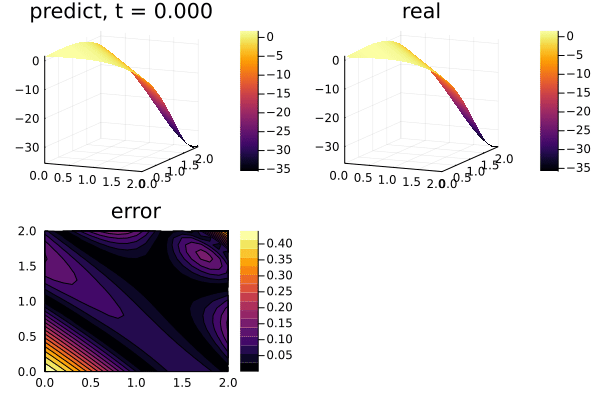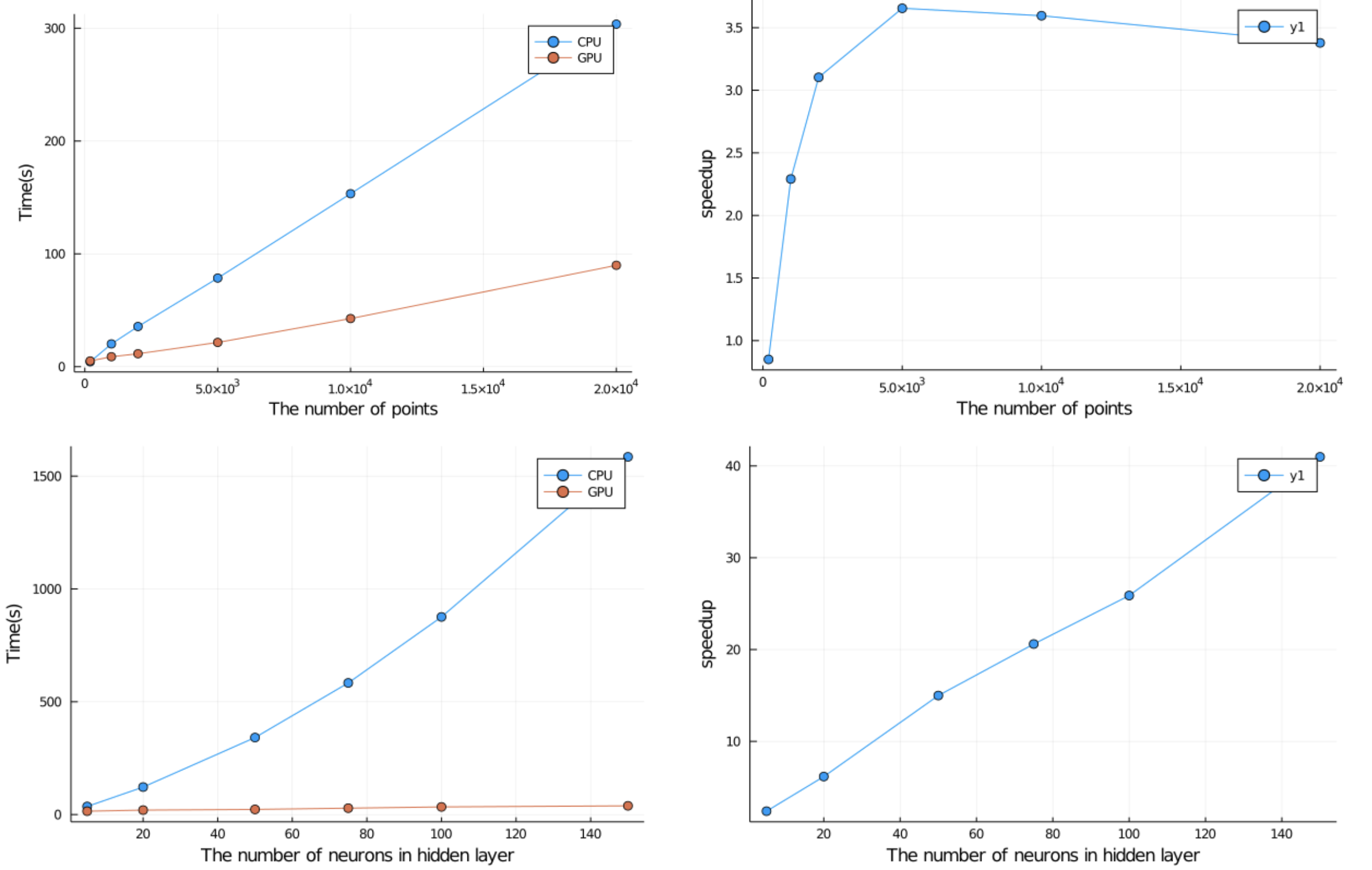# 2-dimensional PDEs with GPU

the 2-dimensional PDE:

$$$∂_t u(x, y, t) = ∂^2_x u(x, y, t) + ∂^2_y u(x, y, t) \, ,$$$

with the initial and boundary conditions:

\begin{align*} u(x, y, 0) &= e^{x+y} \cos(x + y) \, ,\\ u(0, y, t) &= e^{y} \cos(y + 4t) \, ,\\ u(2, y, t) &= e^{2+y} \cos(2 + y + 4t) \, ,\\ u(x, 0, t) &= e^{x} \cos(x + 4t) \, ,\\ u(x, 2, t) &= e^{x+2} \cos(x + 2 + 4t) \, , \end{align*}

on the space and time domain:

$$$x \in [0, 2] \, ,\ y \in [0, 2] \, , \ t \in [0, 2] \, ,$$$

with physics-informed neural networks.

using NeuralPDE, Flux, ModelingToolkit, GalacticOptim, Optim, DiffEqFlux
import ModelingToolkit: Interval, infimum, supremum

@parameters t x y
@variables u(..)
Dxx = Differential(x)^2
Dyy = Differential(y)^2
Dt = Differential(t)
t_min= 0.
t_max = 2.0
x_min = 0.
x_max = 2.
y_min = 0.
y_max = 2.

# 2D PDE
eq  = Dt(u(t,x,y)) ~ Dxx(u(t,x,y)) + Dyy(u(t,x,y))

analytic_sol_func(t,x,y) = exp(x+y)*cos(x+y+4t)
# Initial and boundary conditions
bcs = [u(t_min,x,y) ~ analytic_sol_func(t_min,x,y),
u(t,x_min,y) ~ analytic_sol_func(t,x_min,y),
u(t,x_max,y) ~ analytic_sol_func(t,x_max,y),
u(t,x,y_min) ~ analytic_sol_func(t,x,y_min),
u(t,x,y_max) ~ analytic_sol_func(t,x,y_max)]

# Space and time domains
domains = [t ∈ Interval(t_min,t_max),
x ∈ Interval(x_min,x_max),
y ∈ Interval(y_min,y_max)]

# Neural network
inner = 25
chain = FastChain(FastDense(3,inner,Flux.σ),
FastDense(inner,inner,Flux.σ),
FastDense(inner,inner,Flux.σ),
FastDense(inner,inner,Flux.σ),
FastDense(inner,1))

initθ = CuArray(Float64.(DiffEqFlux.initial_params(chain)))

strategy = GridTraining(0.05)
discretization = PhysicsInformedNN(chain,
strategy;
init_params = initθ)

@named pde_system = PDESystem(eq,bcs,domains,[t,x,y],[u(t, x, y)])
prob = discretize(pde_system,discretization)
symprob = symbolic_discretize(pde_system,discretization)

cb = function (p,l)
println("Current loss is: $l") return false end res = GalacticOptim.solve(prob,ADAM(0.01);cb=cb,maxiters=2500) The remake function allows to rebuild the PDE problem.  prob = remake(prob,u0=res.minimizer) res = GalacticOptim.solve(prob,ADAM(0.001);cb=cb,maxiters=2500) phi = discretization.phi ts,xs,ys = [infimum(d.domain):0.1:supremum(d.domain) for d in domains] u_real = [analytic_sol_func(t,x,y) for t in ts for x in xs for y in ys] u_predict = [first(Array(phi([t, x, y], res.minimizer))) for t in ts for x in xs for y in ys] using Plots using Printf function plot_(res) # Animate anim = @animate for (i, t) in enumerate(0:0.05:t_max) @info "Animating frame$i..."
u_real = reshape([analytic_sol_func(t,x,y) for x in xs for y in ys], (length(xs),length(ys)))
u_predict = reshape([Array(phi([t, x, y], res.minimizer)) for x in xs for y in ys], length(xs), length(ys))
u_error = abs.(u_predict .- u_real)
title = @sprintf("predict, t = %.3f", t)
p1 = plot(xs, ys, u_predict,st=:surface, label="", title=title)
title = @sprintf("real")
p2 = plot(xs, ys, u_real,st=:surface, label="", title=title)
title = @sprintf("error")
p3 = plot(xs, ys, u_error, st=:contourf,label="", title=title)
plot(p1,p2,p3)
end
gif(anim,"3pde.gif", fps=10)
end

plot_(res)## Performance benchmarks

Here are some performance benchmarks for 2d-pde with various number of input points and the number of neurons in the hidden layer, measuring the time for 100 iterations. Сomparing runtime with GPU and CPU.


julia> CUDA.device()
CuDevice(0): Tesla P100-PCIE-16GB